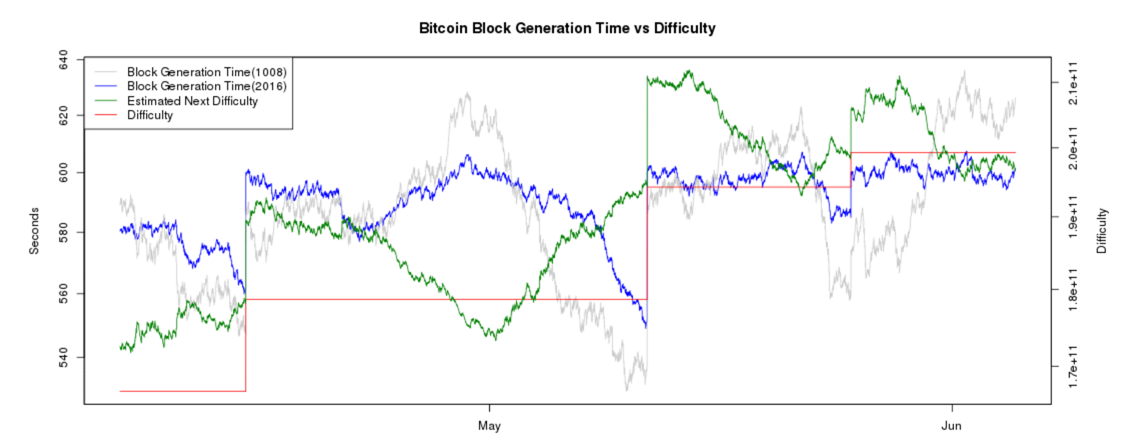# Understanding Bitcoin Difficulty

Bitcoin miners must find a SHA-256 hash that starts with many zeroes. There is no known way to do that except brute-force, adjusting a portion of the input block and calculating hashes over and over until one of them finally fulfills this requirement by chance.

Every 14 days, on average, the Bitcoin difficulty is adjusted, to make the time between blocks stay near 10 minutes.

Real-time data about bitcoin difficulty is posted here:

The chart below shows recent difficulty adjustments (the red line):From the same source, the current Bitcoin difficulty is

``` 199,312,067,531 ```
What does that number mean? The official explanation is very confusing.

A forum post offers this simple formula to convert this difficulty value to bits:

``` log2(difficulty) + 32 ```
So the current difficulty is:
``` log2(199,312,067,531) + 32 = 69.53 bits ```
The latest hash calculated is for Block #414793 with this value:
``` 00000000000000000530216a17ab1e11502720c784975dc7618f8408df6f7c77 ```
Adding spaces every 4 digits makes it easier to count the zeroes:
``` 0000 0000 0000 0000 0530 216a 17ab 1e11 5027 20c7 8497 5dc7 618f 8408 df6f 7c77 ```
There are 17 zeroes, each representing 4 bits, for a total of 68 bits. The next hex-digit is 5, which is less than 8, so there's one additional zero bit for a total of 69 zero bits.

Clearly, that formula works.

## What Does 69.53 Bits Mean?

It means that all the Bitcoin miners added together must calculate a total of 2^69.53 hashes before finding a valid one, on average. And we know that takes approximately 10 minutes. So the average computation rate is:
``` 2^69.53 / 10 min = 1,420,000,000 Giga-hashes per second ```
This is quite close to the actual current hash rate of 1,426,731,353 GH/s.

Posted 6-4-16 by Sam Bowne